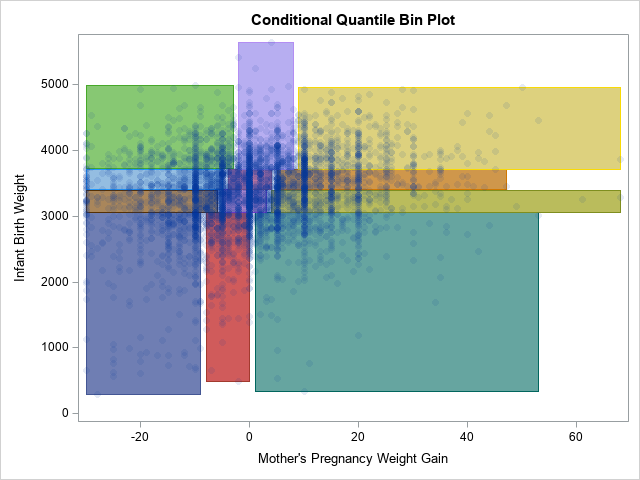A 2-D "bin plot" counts the number of observations in each cell in a regular 2-D grid. The 2-D bin plot is essentially a 2-D version of a histogram: it provides an estimate for the density of a 2-D distribution. As I discuss in the article, "The essential guide to binning in SAS," sometimes you are more interested in displaying bins that contain (approximately) an equal number of observations. This leads to a quantile bin plot.

A SAS programmer on the SAS Support Communities recently requested a variation of the quantile bin plot. In the variation, the bins for the Y variable are computed as usual, but the bins for the X variable are conditional on the Y quantiles. This leads to a situation where the bins do not form a regular grid, but rather vary in both weight and width, as shown to the right. I call this a conditional quantile bin plot. In the graph, there are 12 rectangles. Each contains approximately the same number of observations. The three rectangles across each row contain a quartile of Y values.

This article shows how to construct a conditional quantile bin plot in SAS by first finding the quantiles for the Y variable and then finding the quantiles for the X variable (conditioned on the Y) for each Y quantile. You can solve this problem by using the SAS/IML language (as shown in the Support Community post) or by using PROC RANK and the DATA step, as shown in this article.

Although it is straightforward to compute conditional quantiles, it is less clear how to display the rectangles that represent the cells in the graph. On the Support Community, one solution uses the VECTOR statement in PROC SGPLOT, whereas another solution uses the POLYLINE command in an annotate data set. In this article, I present a third alternative, which is to use a POLYGON statement in PROC SGPLOT. I think that the POLYGON statement is the most powerful option because you can easily display the rectangles as filled, unfilled, in the foreground, in the background, and you can color the polygons in many informative ways.

### Compute the unconditional and conditional quantiles

To compute quantiles, you can use the QNTL function in PROC IML or you can call PROC RANK and use the GROUPS= option. The following statements use PROC RANK. ("KSharp" used this method for his answer on the Support Community.) One noteworthy idea in the following program is to use the CATX or CATT function to form a grouping variable that combines the group names for the X and Y variables.

```%let XVar = MomWtGain; /* name of X variable */ %let YVar = Weight; /* name of Y variable */ %let NumXDiv = 3; /* number of subdivisions (quantiles) for the X variable */ %let NumYDiv = 4; /* number of subdivisions (quantiles) for the Y variable */   /* create the example data */ data Have; set sashelp.bweight(obs=5000 keep=&XVar &YVar); if ^cmiss(&XVar, &YVar); /* only use complete cases */ run;   /* group the Y values into quantiles */ proc rank data=Have out=RankY groups=&NumYDiv ties=high; var &YVar; ranks rank_&YVar; run; proc sort data=RankY; by rank_&YVar; run;   /* Conditioned on each Y quantile, group the X values into quantiles */ proc rank data=RankY out=RankBoth groups=&NumXDiv ties=high; by rank_&YVar; var &XVar; ranks rank_&XVar; run; proc sort data=RankBoth; by rank_&YVar rank_&XVar; run;   /* Trick: Use the CATT fuction to create a group for each unique (RankY, RankX) combination */ data Points; set RankBoth; Group = catt(rank_&YVar, rank_&XVar); run;   proc sgplot data=Points; scatter x=&XVar y=&YVar / group=Group markerattrs=(symbol=CircleFilled) transparency=0.75; /* These REFLINESs are hard-coded for these data. They are the unconditional Y quantiles. */ refline 3061 3402 3716 / axis=Y; run;```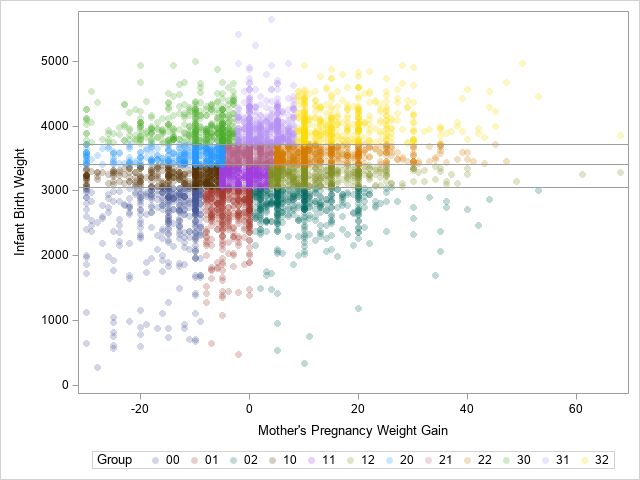The SCATTER statement uses the GROUP= option to color each observation according to the quantile bin to which it belongs. The horizontal reference lines are the 25th, 50th, and 75th percentiles of the Y variable. These lines separate the Y variable into four groups. Within each group, the X variable is divided into three groups. The remainder of the program shows how to construct and plot the 12 rectangles that bound the groups.

### Create polygons for the quantile bins

You can use PROC MEANS and a BY statement to find the minimum and maximum values of the X and Y variables for each cell. As a bonus, PROC MEANS also tells you how many observations are in each cell. If the data are unique values, each cell will have approximately N / 12 observations, where N=5000 is the total number of observations in the data set. If the data have duplicate values, remember that some quantile groups might have more observations than others.

```/* Create polygons from ranks. Each polygon is a rectangle of the form [min(X), max(X)] x [min(Y), max(Y)] */ /* generate min/max for each group. Results are in wide form. */ proc means data=Points noprint; by Group; var &XVar &YVar; output out=Limits(drop=_Type_) min= max= / autoname; run;   proc print data=Limits noobs; run;```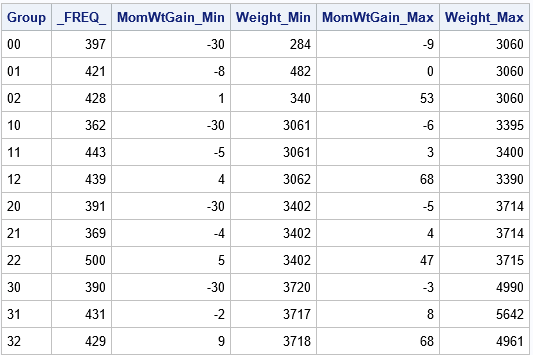For these data, there are between 362 and 500 observations in each cell. If there were no tied values, you would expect 5000/12 ≈ 417 observations in each cell.

The Limits data set contains all the information that you need to construct the cells of the conditional quantile bin plot. PROC MEANS writes the ranges of the variables in a wide format. In order to use the POLYGON statement in PROC SGPLOT, you need to convert the data to long form and use the GROUP variable as an ID variable for the polygon plot:

```/* generate polygons from min/max of groups. Write data in long form. */ data Rectangles; set Limits; xx = &XVar._min; yy = &YVar._min; output; xx = &XVar._max; yy = &YVar._min; output; xx = &XVar._max; yy = &YVar._max; output; xx = &XVar._min; yy = &YVar._max; output; xx = &XVar._min; yy = &YVar._min; output; /* repeat the first obs to close the polygon */ drop &XVar._min &XVar._max &YVar._min &YVar._max; run;   /* combine the data and the polygons. Plot the results */ data All; set Points Rectangles; label _FREQ_ = "Frequency"; run;```

### Create polygons for the quantile bins

The advantage of creating polygons is that you can experiment with many ways to visualize the conditional quantile plot. For example, the simplest quantile plot merely overlays rectangles on a scatter plot of the bivariate data, as follows:

```title "Conditional Quantile Bin Plot"; proc sgplot data=All noautolegend; scatter x=&XVar y=&YVar / markerattrs=(symbol=CircleFilled) transparency=0.9; polygon x=xx y=yy ID=Group; /* plot in the foreground, no fill */ run;```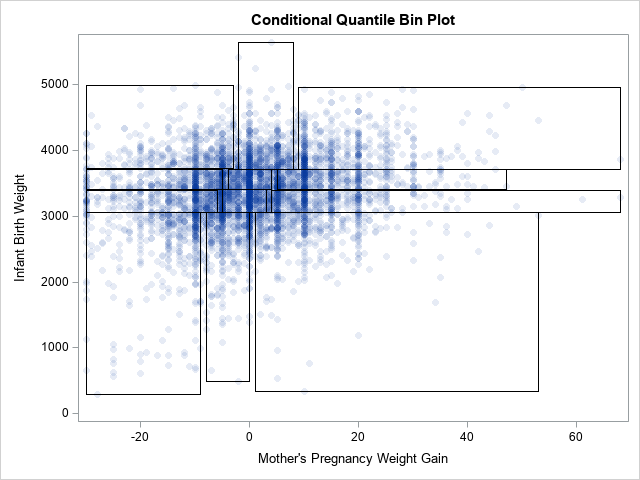On the other hand, you can also display the rectangles in the background and use the GROUP= option to display the rectangles in different colors.

```proc sgplot data=All noautolegend; polygon x=xx y=yy ID=Group / fill outline group=Group; /* background, filled */ scatter x=&XVar y=&YVar / markerattrs=(symbol=CircleFilled) transparency=0.9; run;```

The graph that results from these statements is shown at the top of this article.

To give a third example, you can plot the rectangles in the background and use the COLORRESPONSE= option to color the rectangles according to the number of observations inside each bin. This is shown in the following graph, which uses the default three-color color ramp to assign colors to rectangles.

```proc sgplot data=All; polygon x=xx y=yy ID=Group / fill outline colorresponse=_FREQ_; /* background, filled */ scatter x=&XVar y=&YVar / markerattrs=(symbol=CircleFilled) transparency=0.9; gradlegend; run;```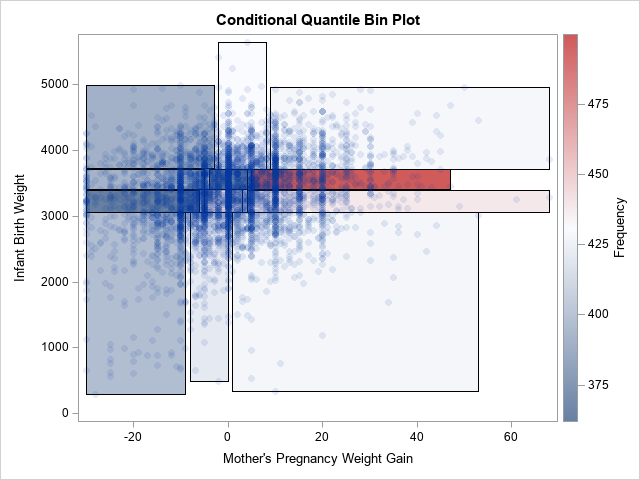### Summary

In summary, this article shows how to construct a 2-D quantile bin plot where the quantiles in the horizontal direction are conditional on the quantiles in the vertical direction. In such a plot, the cells are irregularly spaced but have approximately the same number of observations in each cell. You can use polygons to create the rectangles. The polygons give you a lot of flexibility about how to display the conditional quantile plot.

The post Create a conditional quantile bin plot in SAS appeared first on The DO Loop.The next time you pick up a book, you might want to pause and think about the work that has gone into producing it – and not just from the authors!

The authors of the SAS classic, The Little SAS Book, Sixth Edition, did just that. The Acknowledgement section in the front of a book is usually short – just a few lines to thank family and significant others. In their sixth edition, authors Lora D. Delwiche and Susan J. Slaughter, took it to the next level and produced The Little SAS Book Family Tree. The authors explained:

“Over the years, many people have helped to make this book a reality. We are grateful to everyone who has contributed both to this edition, and to editions in the past. It takes a family to produce a book including reviewers, copyeditors, designers, publishing specialists, marketing specialists, and of course our editors.”### So what happens after you sign a book contract?

First you will be assigned a development editor (DE) who will answer questions and be with you every step of the way – from writing your sample chapter to publication. Your DE will discuss schedules and milestones as well as give you an authoring template, style guidelines, and any software you need to write your book.

Once you have all the resources you need, you'll write your sample chapter. This will help your DE evaluate your writing style, quality of graphics and output, structure, and any potential production issues.

The next step is submitting a draft manuscript for technical review. You'll get feedback from internal and external subject-matter experts, and then you can revise the manuscript based on this feedback. When you and your editor are satisfied with the technical content, your DE will perform a substantive edit on your manuscript, taking particular care with the structure and flow of your writing.

Once in production, your manuscript will be copy edited, and a production specialist will lay out the pages and make sure everything looks good. A graphic designer will work with you to create a cover that encompasses both our branding and your suggestions. Your book will be published in print and e-book versions and made ready for sale.

Finally, after the book is published, a marketing specialist will start promoting your book through our social media channels and other campaigns.

So the next time you pick up a book, spare a thought for the many people who have worked to make it a reality!

How many people does it take to publish a book? was published on SAS Users.Rockin' around the Christmas tree
At the Christmas party hop.
– Brenda Lee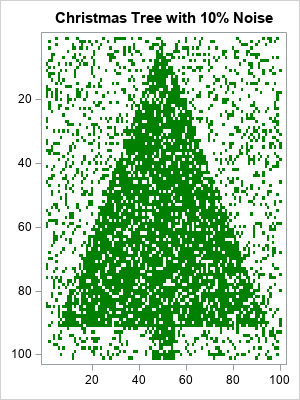Last Christmas, I saw a fun blog post that used optimization methods to de-noise an image of a Christmas tree. Although there are specialized algorithms that remove random noise from an image, I am not going to use a specialized de-noising algorithm. Instead, I will use the SVD algorithm, which is a general-purpose matrix algorithm that has many applications. One application is to construct a low-rank approximation of a matrix. This article applies the SVD algorithm to a noisy image of a Christmas tree. The result is recognizable as a Christmas tree, but much of the noise has been eliminated.

### A noisy Christmas tree image

The image to the right is a heat map of a binary (0/1) matrix that has 101 rows and 100 columns. First, I put the value 1 in certain cells so that the heat map resembles a Christmas tree. About 41% of the cells have the value 1. We will call these cells "the tree".

To add random noise to the image, I randomly switched 10% of the cells. The result is an image that is recognizable as a Christmas tree but is very noisy.

### Apply the SVD to a matrix

As mentioned earlier, I will attempt to de-noise the matrix (image) by using the general-purpose SVD algorithm, which is available in SAS/IML software. If M is the 101 x 100 binary matrix, then the following SAS/IML statements factor the matrix by using a singular-value decomposition. A series plot displays the first 20 singular values (ordered by magnitude) of the noisy Christmas tree matrix:

```call svd(U, D, V, M); /* A = U*diag(D)*V` */ call series(1:20, D) grid={x y} xvalues=1:nrow(D) label={"Component" "Singular Value"};```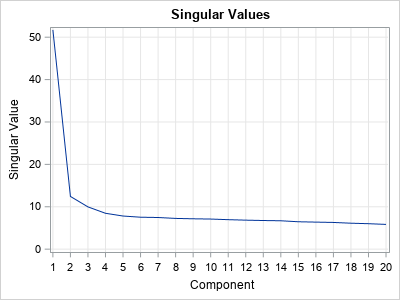The graph (which is similar to a scree plot in principal component analysis) indicates that the first four singular values are somewhat larger than the remainder. The following statements approximate M by using rank-4 matrix. The rank-4 matrix is not a binary matrix, but you can visualize the rank-4 approximation matrix by using a continuous heat map. For convenience, the matrix elements are shifted and scaled into the interval [0,1].

```keep = 4; /* how many components to keep? */ idx = 1:keep; Ak = U[ ,idx] * diag(D[idx]) * V[ ,idx]`; Ak = (Ak - min(Ak)) / range(Ak); /* for plotting, standardize into [0,1] */ s = "Rank " + strip(char(keep)) + " Approximation of Noisy Christmas Tree Image"; call heatmapcont(Ak) colorramp=ramp displayoutlines=0 showlegend=0 title=s range={0, 1};```### Apply a threshold cutoff to classify pixels

The continuous heat map shows a dark image of the Christmas tree surrounded by a light-green "fog". The dark-green pixels represent cells that have values near 1. The light-green pixels have smaller values. You can use a histogram to show the distribution of values in the rank-4 matrix:

```ods graphics / width=400px height=300px; call histogram(Ak);```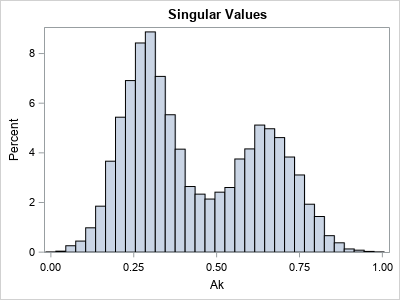You can think of the cell values as being a "score" that predicts whether each cell belongs to the Christmas tree (green) or not (white). The histogram indicates that the values in the matrix appear to be a mixture of two distributions. The high values in the histogram correspond to dark-green pixels (cells); the low values correspond to light-green pixels. To remove the light-green "fog" in the rank-4 image, you can use a "threshold value" to convert the continuous values to binary (0/1) values. Essentially, this operation predicts which cells are part of the tree and which are not.

For every choice of the threshold parameter, there will be correct and incorrect predictions:

• A "true positive" is a cell that belongs to the tree and is colored green.
• A "false positive" is a cell that does not belong to the tree but is colored green.
• A "true negative" is a cell that does not belong to the tree and is colored white.
• A "false negative" is a cell that belongs to the tree but is colored white.

By looking at the histogram, you might guess that a threshold value of 0.5 will do a good job of separating the low and high values. The following statements use 0.5 to convert the rank-4 matrix into a binary matrix, which you can think of as the predicted values of the de-noising process:

```t = 0.5; /* threshold parameter */ s = "Discrete Filter: Threshold = " + strip(char(t,4,2)) ; call HeatmapDisc(Ak > t) colorramp=ramp displayoutlines=0 showlegend=0 title=s;```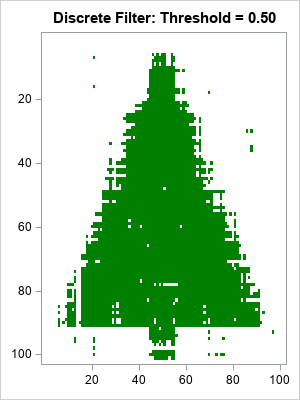Considering that 10% of the original image was corrupted, the heat map of the reconstructed matrix is impressive. It looks like a Christmas tree, but has much less noise. The reconstructed matrix agrees with the original (pre-noise) matrix for 98% of the cells. In addition:

• There are only a few false positives (green dots) that are far from the tree.
• There are some false negatives in the center of the tree, but many fewer than were in the original noisy image.
• The locations where the image is the most ragged is along the edge and trunk of the Christmas tree. In that region, the de-noising could not tell the difference between tree and non-tree.

### The effect of the threshold parameter

You might wonder what the reconstruction would look like for different choices of the cutoff threshold. The following statements create two other heat maps, one for the threshold value 0.4 and the other for the threshold value 0.6. As you might have guessed, smaller threshold values result in more false positives (green pixels away from the tree), whereas higher threshold values result in more false negatives (white pixels inside the tree).

```do t = 0.4 to 0.6 by 0.2; s = "Discrete Filter: Threshold = " + strip(char(t,4,2)) ; call HeatmapDisc(Ak > t) colorramp=ramp displayoutlines=0 showlegend=0 title=s; end;```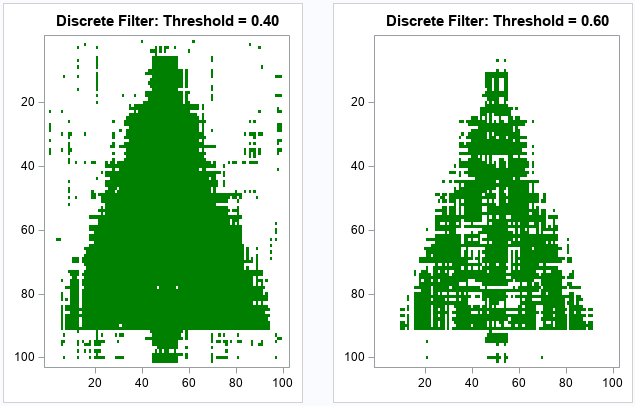### Summary

Although I have presented this experiment in terms of an image of a Christmas tree, it is really a result about matrices and low-rank approximations. I started with a binary 0/1 matrix, and I added noise to 10% of the cells. The SVD factorization enables you to approximate the matrix by using a rank-4 approximation. By using a cutoff threshold, you can approximate the original matrix before the random noise was added. Although the SVD algorithm is a general-purpose algorithm that was not designed for de-noising images, it does a good job eliminating the noise and estimating the original matrix.

If you are interested in exploring these ideas for yourself, you can download the complete SAS program that creates the graphs in this article.

The post Math-ing around the Christmas tree: Can the SVD de-noise an image? appeared first on The DO Loop.Parts 1 and 2 of this blog post discussed exploring and preparing your data using SASPy. To recap, Part 1 discussed how to explore data using the SASPy interface with Python. Part 2 continued with an explanation of how to prepare your data to use it with a machine-learning model. This final installment continues the discussion about preparation by explaining techniques for normalizing your numerical data and one-hot encoding categorical variables.

Some of the numerical features in the examples from parts 1 and 2 have different ranges. The variable Age spans from 0-100 and Hours_per_week from 0-80. These ranges affect the calculation of each feature when you apply them to a supervised learner. To ensure the equal treatement of each feature, you need to scale the numerical features.

The following example uses the SAS STDIZE procedure to scale the numerical features. PROC STDIZE is not a standard procedure available in the SASPy library.  However, the good news is you can add any SAS procedure to SASPy! This feature enables Python users to become a part of the SAS community by contributing to the SASPy library and giving them the chance to use the vast number of powerful SAS procedures. To add PROC STDIZE to SASPy, see the instructions in the blog post Adding SAS procedures to the SASPy interface to Python.

After you add the STDIZE to SASPy, run the following code to scale the numerical features. The resulting values will be between 0 and 1.

```# Creating a SASPy stat objcect stat = sas.sasstat()```
```# Use the stdize function that was added to SASPy to scale our features stat_result = stat.stdize(data=cen_data_logTransform, procopts = 'method=range out=Sasuser.afternorm', var = 'age education_num capital_gain capital_loss hours_per_week')```

To use the STDIZE procedure in SASPy we need to specify the method and the output data set in the statement options. For this we use the "procopts" option and we specify range as our method and our "out" option to a new SAS data set, afternorm.

After running the STDIZE procedure we assign the new data set into a SAS data object.

`norm_data = sas.sasdata('afternorm', libref='SASuser')`

Now let's verify if we were successful in transforming our numerical features

`norm_data.head(obs=5)`

The output looks great! You have normalized the numerical features. So, it's time to tackle the last data-preparation step.

## One-Hot Encoding

Now that you have adjusted the numerical features, what do you do with the categorical features? The categories Relationship, Race, Sex, and so on are in string format. Statistical models cannot interpret these values, so you need to transform the values from strings into a numerical representation. The one-hot encoding process provides the transformation you need for your data.

To use one-hot encoding, use the LOGISTIC procedure from the SASPy Stat class. SASPy natively includes the LOGISTIC procedure, so you can go straight to coding. To generate the syntax for the code below, I followed the instructions from Usage Note 23217: Saving the coded design matrix of a model to a data set.

```stat_proc_log = stat.logistic(data=norm_data, procopts='outdesign=SASuser.test1 outdesignonly', cls = "workclass education_level marital_status occupation relationship race sex native_country / param=glm", model = "age = workclass education_level marital_status occupation relationship race sex native_country / noint")```

To view the results from this code, create a SAS data object from the newly created data set, as shown in this example:

```one_hot_data = sas.sasdata('test1', libref='SASuser') display(one_hot_data.head(obs=5))```

The output: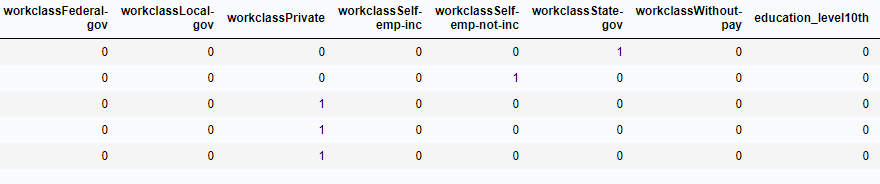Our data was successfully one-hot encoded! For future reference, due to SAS’ analytical power, this step is not required. When including a categorical feature in a class statement the procedure automatically generates a design matrix with the one-hot encoded feature. For more information, I recommend reading this post about different ways to create a design matrix in SAS.

## Finally

You made it to the end of the journey! I hope everyone who reads these blogs can see the value that SASPy brings to the machine-learning community. Give SASPy  a try, and you'll see the power it can bring to your SAS solutions.

Stay curious, keep learning, and (most of all) continue innovating.Bringing the power of SAS to your Python scripts can be a game changer. An easy way to do that is by using SASPy, a Python interface to SAS allowing Python developers to use SAS® procedures within Python. However, not all SAS procedures are included in the SASPy library. So, what do you do if you want to use those excluded procedures? Easy! The SASPy library contains functionality enabling you to add SAS procedures to the SASPy library. In this post, I'll explain the process.

The basics for adding procedures are covered in the Contributing new methods section in the SASPy documentation. To further assist you, this post expands upon the steps, providing step-by-step details for adding the STDIZE procedure to SASPy. For a hands-on application of the use case refer the blog post Machine Learning with SASPy: Exploring and Preparing your data - Part 3.

This is your chance to contribute to the project! Whereas, you can choose to follow the steps below as a one-off solution, you also have the choice to share your work and incorporate it in the SASPy repository.

## Prerequisites

Before you add a procedure to SASPy, you need to perform these prerequisite steps:

1. Identify the SAS product associated with the procedure you want to add, e.g. SAS/STAT, SAS/ETS, SAS Enterprise Miner, etc.
2. Locate the SASPy file (for example, sasstat.py, sasets.py, and so on) corresponding to the product from step 1.
3. Ensure you have a current license for the SAS product in question.

## Adding a SAS procedure to SASPy

SASPy utilizes Python Decorators to generate the code for adding SAS procedures. Roughly, the process is:

1. define the procedure
2. generate the code to add
3. add the code to the proper SASPy file
4. (optional)create a pull request to add the procedure to the SASPy repository

Below we'll walk through each step in detail.

### Create a set of valid statements

Start a new python session with Jupyter and create a list of valid arguments for the chosen procedure. You determine the arguments for the procedure by searching for your procedure in the appropriate SAS documentation. For example, the PROC STDIZE arguments are documented in the SAS/STAT® 15.1 User's Guide, in the The STDIZE Procedure section, with the contents:

For example, I submitted the following command to create a set of valid arguments for PROC STDIZE:

`lset = {'STDIZE', 'BY', 'FREQ', 'LOCATION', 'SCALE', 'VAR', 'WEIGHT'}`

### Call the doc_convert method

The doc_convert method takes two arguments: a list of valid statements (method_stmt) and the procedure name (stdize).

```import saspy   print(saspy.sasdecorator.procDecorator.doc_convert(lset, 'STDIZE')['method_stmt']) print(saspy.sasdecorator.procDecorator.doc_convert(lset, 'STDIZE')['markup_stmt'])```

The command generates the method call and the docstring markup like the following:

```def STDIZE(self, data: [SASdata', str] = None, by: [str, list] = None, location: str = None, scale: str = None, stdize: str = None, var: str = None, weight: str = None, procopts: str = None, stmtpassthrough: str = None, **kwargs: dict) -> 'SASresults': Python method to call the STDIZE procedure.   Documentation link:   :param data: SASdata object or string. This parameter is required. :parm by: The by variable can be a string or list type. :parm freq: The freq variable can only be a string type. :parm location: The location variable can only be a string type. :parm scale: The scale variable can only be a string type. :parm stdize: The stdize variable can be a string type. :parm var: The var variable can only be a string type. :parm weight: The weight variable can be a string type. :parm procopts: The procopts variable is a generic option avaiable for advanced use It can only be a string type. :parm stmtpassthrough: The stmtpassthrough variable is a generic option available for advanced use. It can only be a string type. :return: SAS Result Object```

### Update SASPy product file

We'll take the output and add it to the appropriate product file (sasstat.py in this case). When you open this file, be sure to open it with administrative privileges so you can save the changes. Prior to adding the code to the product file, perform the following tasks:

1. add @procDecorator.proc_decorator({}) before the function definition
2. add the proper documentation link from the SAS Programming Documentation site
3. add triple quotes ("""") to comment out the second section of code

The following output shows the final code to add to the sasstat.py file:

```@procDecorator.proc_decorator({}) def STDIZE(self, data: [SASdata', str] = None, by: [str, list] = None, location: str = None, scale: str = None, stdize: str = None, var: str = None, weight: str = None, procopts: str = None, stmtpassthrough: str = None, **kwargs: dict) -> 'SASresults': """ Python method to call the STDIZE procedure.   Documentation link: https://go.documentation.sas.com/?cdcId=pgmsascdc&cdcVersion=9.4_3.4&docsetId=statug&docsetTarget=statug_stdize_toc.htm&locale=en :param data: SASdata object or string. This parameter is required. :parm by: The by variable can be a string or list type. :parm freq: The freq variable can only be a string type. :parm location: The location variable can only be a string type. :parm scale: The scale variable can only be a string type. :parm stdize: The stdize variable can be a string type. :parm var: The var variable can only be a string type. :parm weight: The weight variable can be a string type. :parm procopts: The procopts variable is a generic option avaiable for advanced use It can only be a string type. :parm stmtpassthrough: The stmtpassthrough variable is a generic option available for advanced use. It can only be a string type. :return: SAS Result Object """```

### Update sasdecorator file with the new method

Alter the sasdecorator.py file by adding stdize in the code on line 29, as shown below.

`if proc in ['hplogistic', 'hpreg', 'stdize']:`

Important: The update to the sasdecorator file is only a requirement when you add a procedure with no plot options. The sasstat.py library assumes all procedures produce plots. However, PROC STDIZE does not include them. So, you should perform this step ONLY when your procedure does not include plot options. This will more than likely change in a future release, so please follow the Github page for any updates.

### Document a test for your function

Make sure you write at least one test for the procedure. Then, add the test to the appropriate testing file.

## Finally

Congratulations! All done. You now have the knowledge to add even more procedures in the future.

After you add your procedure, I highly recommend you contribute your procedure to the SASPy GitHub library. To contribute, follow the outlined instructions on the Contributing Rules GitHub page.

Adding SAS® procedures to the SASPy interface to Python was published on SAS Users.Binary matrices are used for many purposes. I have previously written about how to use binary matrices to visualize missing values in a data matrix. They are also used to indicate the co-occurrence of two events. In ecology, binary matrices are used to indicate which species of an animal are present in which ecological site. For example, if you remember your study of Darwin's finches in high school biology class, you might recall that certain finches (species) are present or absent on various Galapagos islands (sites). You can use a binary matrix to indicate which finches are present on which islands.

Recently I was involved in a project that required performing a permutation test on rows of a binary matrix. As part of the project, I had to solve three smaller problems involving rows of a binary matrix:

1. Given two rows, find the locations at which the rows differ.
2. Given two binary matrices, determine how similar the matrices are by computing the proportion of elements that are the same.
3. Given two rows, swap some of the elements that differ.

This article shows how to solve each problem by using the SAS/IML matrix language. A future article will discuss permutation tests for binary matrices. For clarity, I introduce the following macro that uses a temporary variable to swap two sets of values:

```/* swap values of A and B. You can use this macro in the DATA step or in the SAS/IML language */ %macro SWAP(A, B, TMP=_tmp); &TMP = &A; &A = &B; &B = &TMP; %mend;```

### Where do binary matrices differ?

The SAS/IML matrix language enables you to treat matrices as high-level objects. You often can answer questions about matrices without writing loops that iterate over the elements of the matrices. For example, if you have two matrices of the same dimensions, you can determine the cells at which the matrices are unequal by using the "not equal" (^=) operator. The following SAS/IML statements define two 2 x 10 matrices and use the "not equal" operator to find the cells that are different:

```proc iml; A = {0 0 1 0 0 1 0 1 0 0 , 1 0 0 0 0 0 1 1 0 1 }; B = {0 0 1 0 0 0 0 1 0 1 , 1 0 0 0 0 1 1 1 0 0 }; colLabel = "Sp1":("Sp"+strip(char(ncol(A)))); rowLabel = "A1":("A"+strip(char(nrow(A))));   /* 1. Find locations where binary matrices differ */ Diff = (A^=B); print Diff[c=colLabel r=rowLabel];```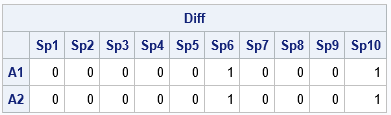The matrices A and B are similar. They both have three 1s in the first row and fours 1s in the second row. However, the output shows that the matrices are different for the four elements in the sixth and tenth columns. Although I used entire matrices for this example, the same operations work on row vectors.

### The proportion of elements in common

You can use various statistics to measure the similarity between the A and B matrices. A simple statistic is the proportion of elements that are in common. These matrices have 20 elements, and 16/20 = 0.8 are common to both matrices. You can compute the proportion in common by using the express (A=B)[:], or you can use the following statements if you have previously computed the Diff matrix:

```/* 2. the proportion of elements in B that are the same as in A */ propDiff = 1 - Diff[:]; print propDiff;```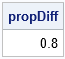As a reminder, the mean subscript reduction operator ([:]) computes the mean value of the elements of the matrix. For a binary matrix, the mean value is the proportion of ones.

### Swap elements of rows

The first two tasks were easy. A more complicated task is swapping values that differ between rows. The swapping operation is not difficult, but it requires finding the k locations where the rows differ and then swapping all or some of those values. In a permutation test, the number of elements that you swap is a random integer between 1 and k, but for simplicity, this example uses the SWAP macro to swap two cells that differ. For clarity, the following example uses temporary variables such as x1, x2, d1, and d2 to swap elements in the matrix A:

```/* specify the rows whose value you want to swap */ i1 = 1; /* index of first row to compare and swap */ i2 = 2; /* index of second row to compare and swap */   /* For clarity, use temp vars x1 & x2 instead of A[i1, ] and A[i2, ] */ x1 = A[ i1, ]; /* get one row */ x2 = A[ i2, ]; /* get another row */ idx = loc( x1^=x2 ); /* find the locations where rows differ */ if ncol(idx) > 0 then do; /* do the rows differ? */ d1 = x1[ ,idx]; /* values at the locations that differ */ d2 = x2[ ,idx]; print (d1//d2)[r={'r1' 'r2'} L='Original'];   /* For a permutation test, choose a random number of locations to swap */ /* numSwaps = randfun(1, "Integer", 1, n); idxSwap = sample(1:ncol(idx), numSwaps, "NoReplace"); */ idxSwap = {2 4}; /* for now, hard-code locations to swap */ %SWAP(d1[idxSwap], d2[idxSwap]); print (d1//d2)[r={'d1' 'd2'} L='New']; /* update matrix */ A[ i1, idx] = d1; A[ i2, idx] = d2; end; print A[c=colLabel r=rowLabel];```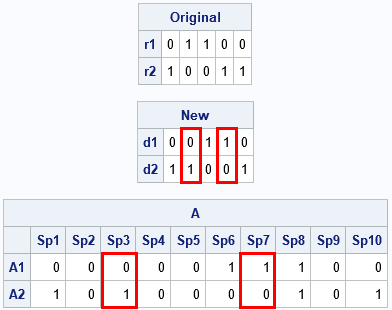The vectors x1 and x2 are the rows of A to compare. The vectors d1 and d2 are the subvectors that contain only the elements of x1 and x2 that differ. The example swaps the second and fourth columns of d1 and d2. The new values are then inserted back into the matrix A. You can compare the final matrix to the original to see that the process swapped two elements in each of two rows.

Although the examples are for binary matrices, these techniques work for any numerical matrices.

The post Swap elements in binary matrices appeared first on The DO Loop.View more Problem Solvers posts

The DATA step has been around for many years and regardless of how many new SAS® products and solutions are released, the DATA step remains a popular way to create and manipulate SAS data sets. The SAS language contains a wide variety of approaches that provide an endless number of ways to accomplish a goal. Whether you are reshaping a data set entirely or simply assigning values to a new variable, there are numerous tips and tricks that you can use to save time and keystrokes. Here are a few that Technical Support offers to customers on a regular basis.

## Writing file contents to the SAS® log

Perhaps you are reading a file and seeing “invalid data” messages in the log or you are not sure which delimiter is used between variables. You can use the null INPUT statement to read a small sample of the data. Specify the amount of data that you want to read by adjusting options in the INFILE statement. This example reads one record with 500 bytes:

``` data _null_; infile 'path' recfm=f lrecl=500 obs=1; input; list; run;```

The LIST statement writes the current record to the log. In addition, it includes a ruled line above the record so that it is easier to see the data for each column of the file. If the data contains at least one unprintable character, you will see three lines of output for each record written.

For example:

```RULE: ----+----1----+----2----+----3----+----4----+----5----+----6----+   1 CHAR this is a test.123.dog.. 24 ZONE 766726726276770333066600 NUMR 48930930104534912394F7DA```

The line beginning with CHAR describes the character that is represented by the two hexadecimal characters underneath. When you see a period in that line, it might actually be a period, but it often means that the hexadecimal characters do not map to a specific key on the keyboard. Also, column 15 for this data is a tab ('09'x). By looking at this record in the log, you can see that the first variable contains spaces and that the file is tab delimited.

## Writing hexadecimal output for all input

Since SAS® 9.4M6 (TS1M6), the HEXLISTALL option in the LIST statement enables all lines of input data to be written in hexadecimal format to the SAS log, regardless of whether there are unprintable characters in the data:

` data mylib.new / hexlistall;`

## Removing “invalid data” messages from the log

When SAS reads data into a data set, it is common to encounter values that are invalid based on the variable type or the informat that is specified for a variable. You should examine the SAS log to assess whether the informat is incorrect or if there are some records that are actually invalid. After you ensure that the data is being read correctly, you can dismiss any “invalid data” messages by using double question mark (??) modifiers after the variable name in question:

` input date ?? mmddyy10.;`

You can also use the question mark modifiers when you convert a character variable to numeric with the INPUT function:

` newdate=input(olddate,?? mmddyy10.);`

The above syntax reads all values with the MMDDYY10. informat and then dismisses the notes to the log when some values for the OLDDATE variable are invalid.

## Sharing files between UNIX and Microsoft Windows operating systems

The end-of-record markers in text files are different for UNIX and Windows operating systems. If you needed to share a file between these systems, the file used to need preprocessing in order to change the markers to the desired type or perhaps specify a delimiter. Now, the TERMSTR= option in the INFILE statement enables you to specify the end-of-record marker in the incoming file.

If you are working in a UNIX environment and you need to read a file that was created in a Windows environment, use the TERMSTR=CRLF option:

` infile 'file-specification' termstr=crlf ;`

If you are in a Windows environment and you need to read a file that was created in a UNIX environment, use this syntax:

` infile 'file-specification' termstr=lf ;`

## Adapting array values from an ARRAY statement

The VNAME function makes it very convenient to use the variable names from an ARRAY statement. You most often use an ARRAY statement to obtain the values from numerous variables. In this example, the SQL procedure makes it easy to store the unique values of the variable Product into the macro variable &VARLIST and that number of values into the macro variable &CT (another easy tip). Within the DO loop, you obtain the names of the variables from the array, and those values from the array then become variable names.

``` proc sql noprint; select distinct(product) into :varlist separated by ' ' from one; select count(distinct product) into :ct from one; quit;   …more DATA step statements… array myvar(&ct) \$ &varlist; do i=1 to &ct; if product=vname(myvar(i)) then do; myvar(i)=left(put(contract,8.)); end; …more DATA step statements…```

## Using a mathematical equation in a DO loop

A typical DO loop has a beginning and an end, both represented by integers. Did you know you can use an equation to process data more easily? Suppose that you want to process every four observations as one unit. You can run code similar to the following:

``` …more DATA step statements… J+1; do i=((j*4)-3) to (j*4); set data-set-name point=I; …more DATA step statements… end;```

## Using an equation to point to an array element

With small data sets, you might want to put all values for all observations into a single observation. Suppose that you have a data set with four variables and six observations. You can create an array to hold the existing variables and also create an array for the new variables. Here is partial code to illustrate how the equation dynamically points to the correct new variable name in the array:

``` array old(4) a b c d; array test (24) var1-var24; retain var1-var24; do i=1 to nobs; set w nobs=nobs point=i; do j=1 to 4; test(((i-1)*4)+j)=old(j); end; end;```

## Creating a hexadecimal reference chart

How often have you wanted to know the hexadecimal equivalent for a character or vice versa? Sure, you can look up a reference chart online, but you can also create one with a short program. The BYTE function returns values in your computer’s character set. The PUT statement writes the decimal value, hexadecimal value, and equivalent character to the SAS log:

``` data a; do i=0 to 255; x=byte(i); put i=z3. i=hex2. x=; end; run;```

When the resulting character is unprintable, such as a carriage return and line feed (CRLF) character, the X value is blank.

## Conclusion

Hopefully, these new tips will be useful in your future programming. If you know some additional tips, please comment them below so that readers of this blog can add even more DATA step tips to their arsenal! If you would like to learn more about DATA step, check out these other blogs:

Old reliable: DATA step tips and tricks was published on SAS Users.View more Problem Solvers posts

The DATA step has been around for many years and regardless of how many new SAS® products and solutions are released, the DATA step remains a popular way to create and manipulate SAS data sets. The SAS language contains a wide variety of approaches that provide an endless number of ways to accomplish a goal. Whether you are reshaping a data set entirely or simply assigning values to a new variable, there are numerous tips and tricks that you can use to save time and keystrokes. Here are a few that Technical Support offers to customers on a regular basis.

## Writing file contents to the SAS® log

Perhaps you are reading a file and seeing “invalid data” messages in the log or you are not sure which delimiter is used between variables. You can use the null INPUT statement to read a small sample of the data. Specify the amount of data that you want to read by adjusting options in the INFILE statement. This example reads one record with 500 bytes:

``` data _null_; infile 'path' recfm=f lrecl=500 obs=1; input; list; run;```

The LIST statement writes the current record to the log. In addition, it includes a ruled line above the record so that it is easier to see the data for each column of the file. If the data contains at least one unprintable character, you will see three lines of output for each record written.

For example:

```RULE: ----+----1----+----2----+----3----+----4----+----5----+----6----+   1 CHAR this is a test.123.dog.. 24 ZONE 766726726276770333066600 NUMR 48930930104534912394F7DA```

The line beginning with CHAR describes the character that is represented by the two hexadecimal characters underneath. When you see a period in that line, it might actually be a period, but it often means that the hexadecimal characters do not map to a specific key on the keyboard. Also, column 15 for this data is a tab ('09'x). By looking at this record in the log, you can see that the first variable contains spaces and that the file is tab delimited.

## Writing hexadecimal output for all input

Since SAS® 9.4M6 (TS1M6), the HEXLISTALL option in the LIST statement enables all lines of input data to be written in hexadecimal format to the SAS log, regardless of whether there are unprintable characters in the data:

` data mylib.new / hexlistall;`

## Removing “invalid data” messages from the log

When SAS reads data into a data set, it is common to encounter values that are invalid based on the variable type or the informat that is specified for a variable. You should examine the SAS log to assess whether the informat is incorrect or if there are some records that are actually invalid. After you ensure that the data is being read correctly, you can dismiss any “invalid data” messages by using double question mark (??) modifiers after the variable name in question:

` input date ?? mmddyy10.;`

You can also use the question mark modifiers when you convert a character variable to numeric with the INPUT function:

` newdate=input(olddate,?? mmddyy10.);`

The above syntax reads all values with the MMDDYY10. informat and then dismisses the notes to the log when some values for the OLDDATE variable are invalid.

## Sharing files between UNIX and Microsoft Windows operating systems

The end-of-record markers in text files are different for UNIX and Windows operating systems. If you needed to share a file between these systems, the file used to need preprocessing in order to change the markers to the desired type or perhaps specify a delimiter. Now, the TERMSTR= option in the INFILE statement enables you to specify the end-of-record marker in the incoming file.

If you are working in a UNIX environment and you need to read a file that was created in a Windows environment, use the TERMSTR=CRLF option:

` infile 'file-specification' termstr=crlf ;`

If you are in a Windows environment and you need to read a file that was created in a UNIX environment, use this syntax:

` infile 'file-specification' termstr=lf ;`

## Adapting array values from an ARRAY statement

The VNAME function makes it very convenient to use the variable names from an ARRAY statement. You most often use an ARRAY statement to obtain the values from numerous variables. In this example, the SQL procedure makes it easy to store the unique values of the variable Product into the macro variable &VARLIST and that number of values into the macro variable &CT (another easy tip). Within the DO loop, you obtain the names of the variables from the array, and those values from the array then become variable names.

``` proc sql noprint; select distinct(product) into :varlist separated by ' ' from one; select count(distinct product) into :ct from one; quit;   …more DATA step statements… array myvar(&ct) \$ &varlist; do i=1 to &ct; if product=vname(myvar(i)) then do; myvar(i)=left(put(contract,8.)); end; …more DATA step statements…```

## Using a mathematical equation in a DO loop

A typical DO loop has a beginning and an end, both represented by integers. Did you know you can use an equation to process data more easily? Suppose that you want to process every four observations as one unit. You can run code similar to the following:

``` …more DATA step statements… J+1; do i=((j*4)-3) to (j*4); set data-set-name point=I; …more DATA step statements… end;```

## Using an equation to point to an array element

With small data sets, you might want to put all values for all observations into a single observation. Suppose that you have a data set with four variables and six observations. You can create an array to hold the existing variables and also create an array for the new variables. Here is partial code to illustrate how the equation dynamically points to the correct new variable name in the array:

``` array old(4) a b c d; array test (24) var1-var24; retain var1-var24; do i=1 to nobs; set w nobs=nobs point=i; do j=1 to 4; test(((i-1)*4)+j)=old(j); end; end;```

## Creating a hexadecimal reference chart

How often have you wanted to know the hexadecimal equivalent for a character or vice versa? Sure, you can look up a reference chart online, but you can also create one with a short program. The BYTE function returns values in your computer’s character set. The PUT statement writes the decimal value, hexadecimal value, and equivalent character to the SAS log:

``` data a; do i=0 to 255; x=byte(i); put i=z3. i=hex2. x=; end; run;```

When the resulting character is unprintable, such as a carriage return and line feed (CRLF) character, the X value is blank.

## Conclusion

Hopefully, these new tips will be useful in your future programming. If you know some additional tips, please comment them below so that readers of this blog can add even more DATA step tips to their arsenal! If you would like to learn more about DATA step, check out these other blogs:

Old reliable: DATA step tips and tricks was published on SAS Users.